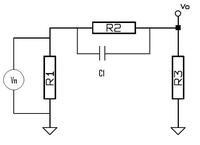# How can the output noise of a circuit which contains Capacitance?

Status
Not open for further replies.

#### Mansour_M

##### Full Member level 1
Consider the circuit in the attached picture.

R2 and R3 are noiseless. and V1 = (4*k*T*R1*B)

if C1 was not in the circuit, it would be possible to use the following equation and calculate the output noise voltage.

V0 = R3*V1/(R1 + R2 + R3)

However I do not know how to calculate the output noise if C1 exists.

In the following link, the noise analysis of a RC is explained. In the text a "Brick Wall" technique is described. I have no idea how to use this technique and I could not find any reference for that.

http://jcatsc.com/media/TechnicalReports/17-Noise2.pdfThermal noise is generated only by resistors. Capacitors only affect the frequency spectrum of it.

Your circuit will generate noise voltage like one resistor replacing R1,R2 and R3. The source Vin can also have its internal resistance, this must be included in parallel to R1.

With the capacitor you generate the loop attenuation with phasors to account for the capacitance. The voltage output will then be a function of frequency. If you want the total voltage output noise then you will have to integrate that function over the bandwidth of interest.

Status
Not open for further replies.